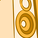# App Development

Announcements
Qlik Insider: SaaS capabilities for Data Integration and Analytics, May 25th! Register Now
cancel
Showing results for
Did you mean:## Obtain the latest Year - Sum Quantity Sold

Hi - I am trying to create a variable that does the following:

• Data contains Sales Date for the past four years (2016 - 2019) and the quantity sold
• I would like have a variable which calculates the sum of the quantity sold for the most recent year (hence 2019)

Table fields:

SalesDate

QuantitySold

Variable:

vYearMaxQuantitySold:

=Sum({\$<[SalesDate.autoCalendar.Year] = {\$(=max([SalesDate.autoCalendar.Year]))}>}[QuantitySold])

I try the formula above - does not work - any thoughts ? Jerry

1 Solution

Accepted SolutionsMVP

Try like this

vYearMaxQuantitySold

Sum({\$<[SalesDate.autoCalendar.Year] = {\$(=max([SalesDate.autoCalendar.Year]))}>}[QuantitySold])

And use in the chart like \$(vYearMaxQuantitySold)

In the variable declaration, don't use = sign. Then it calculated the value n store in the variable. So if u use the variable in the chart, result might be wrong.

Thanks & Regards,
Mayil Vahanan R
2 RepliesAnonymous
Not applicable

Variable:

vYearMaxQuantitySold =max([SalesDate.autoCalendar.Year])

=Sum({\$<[SalesDate.autoCalendar.Year] = {\$(vYearMaxQuantitySold)}>}[QuantitySold])MVP

Try like this

vYearMaxQuantitySold

Sum({\$<[SalesDate.autoCalendar.Year] = {\$(=max([SalesDate.autoCalendar.Year]))}>}[QuantitySold])

And use in the chart like \$(vYearMaxQuantitySold)

In the variable declaration, don't use = sign. Then it calculated the value n store in the variable. So if u use the variable in the chart, result might be wrong.

Thanks & Regards,
Mayil Vahanan R